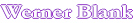Home            PROGRAM TO CALCULATE THE VISCOSITY OF POLYMER BLENDS This program utilizes the WLF equation to calculate the glass transition temperature (Tg) of a polymer, oligomer or an solution. This information is used in conjunction with the FOX equation to obtain the Tg of a polymer blend and the resulting viscosity of the blend. WLF equation Visc(log)= C- A(T-Tg)/(B+T-Tg) This progam uses a modification of this equation, which uses universal constants according to Nielsen. These universal constants are reasonable accurate for most polymers. If a deviation is found for a series of polymers these constants can be readjusted. The Fox equation is normally used to calculate the Tg of acrylic polymers from the Tg of the individual monomers, but it can also be modified to be used calculating the Tg of polymer or oligomer blends.. No unusual interaction between the polymers is assumed. 1/Tgco=Wf1/Tg1+Wf2/Tg2+Wfx/Tgx        Tgco= Tg polymer blend, Wfx=weight fraction polymer x, Tgx=Tg polymer  x As input the viscosity, solids and temperature of the polymer solution is required. Hydroxyl numbers of the blend can also be calculated. INPUT MEASURED DATA Solids Viscosity Temperature OH number Solvent. WLF EQUATION % CPS °C as supplied % Constants (Nielsen) Polymer composition 1 C= Polymer composition 2 A= Polymer composition 3 B= Polymer composition 4 Input Polymer Blend Polymer Solvent Hydroxyl Polymer composition 1 Polymer composition 2 Polymer composition 3 Polymer composition 4 Total Calculate Viscosity Polymer Blend Temperature, °C Delta Nonvolatile, % Hydroxyl number Temperature,  °C Viscosity, Poise Viscosity, cps M.L.Williams, R.F.Landel and  J.D.Ferry, J.Am.Chem.Soc.77,3701(1955) L.E.Nielsen, Polymer Rheology, Marcel Dekker, 1977,pp.33,74,133

Automatic recalculation

 Last edited on: October 20, 2010 Copyright®, Design, Layout and Technical Content by: